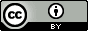## Sunday, September 23, 2012

### The chemical potentials are equal at equilibrium

This is Figure 10.1 from Dill and Bromberg's Molecular Driving Forces, which is my favorite book on statistical mechanics. It is a beautiful example from a beautiful book.

The chemical potential of a species is defined as the change in free energy with respect to the number of molecules of that species, when temperature, volume, and the number of other species are all kept constant:$$\mu_I=\left(\frac{\partial A}{\partial N_I}\right)_{V,T,N_{J\ne I}}$$Let's explore this for the simple four-bead polymer shown in the figure above.  In a previous post I derived the expression for the free energy:$$A=N\varepsilon_0 p_{uf}+TNk\left( p_f\ln(p_f)+p_{uf}\ln\left(\frac{p_{uf}}{4}\right) \right)\\A=\varepsilon_0 N_{uf}+Tk\left( N_f\ln\left(\frac{N_{f}}{N_{f}+N_{uf}}\right)+N_{uf}\ln\left(\frac{N_{uf}}{4(N_{f}+N_{uf})}\right)\right)$$Starting from the latter expression finding the chemical potentials for the folded and unfolded macrostates are: $$\mu_{f}=\left(\frac{\partial A}{\partial N_f}\right)_{V,T,N_{uf}}=kT\ln(p_f)$$ $$\mu_{uf}=\left(\frac{\partial A}{\partial N_{uf}}\right)_{V,T,N_{f}}=\varepsilon_0+kT\ln\left(\frac{p_{uf}}{4}\right)$$The free energy is the sum of the chemical potentials$$A=N_f\mu_f+N_{uf}\mu_{uf}$$Keeping in mind that $N_f=Np_f$ and $N_{uf}=Np_{uf}$ I hope it is obvious to you that this is true.

The chemical potentials are equal at equilibrium
If temperature and volume are constant the change in free energy is given by:$$dA=\mu_fdN_f+\mu_{uf}dN_{uf}$$At equilibrium $dA=0$ and the total number of particles is constant: $dN_f=-dN_{uf}$. Therefore$$\mu_f=\mu_{uf}$$which reduces to the Boltzmann equilibrium distribution:$$kT\ln(p_f)=\varepsilon_0+kT\ln\left(\frac{p_{uf}}{4}\right)\\ \frac{p_{uf}}{p_f}=e^{-( \varepsilon_0-kT\ln(4))/kT}$$The equilibrium constant
The ratio of probabilities is usually referred to as the equilibrium constant $K$:$$K=\frac{p_{uf}}{p_f}$$although it is usually written in terms of concentrations:$$K=\frac{p_{uf}}{p_f}=\frac{N_{uf}}{N_f}=\frac{N_{uf}/V}{N_{f}/V}=\frac{[uf]}{[f]}$$However, this is technically incorrect for two reasons.  One reason is that it doesn't work in general.  For example:$$\frac{p_ip_j}{p_k}\ne\frac{N_{i}/V N_{j}/V}{N_{k}/V}$$To get around this I write:$$K=\frac{[uf]/[c]^\ominus}{[f]/[c]^\ominus}$$where $[c]^\ominus$ is a standard state concentration, usually defined as 1 molar or 1 molal. Another reason is that when I use concentrations I assume that all polymer molecules are either folded or unfolded, whereas in real solutions some polymers might be stuck together.  To correct for this I introduce activity coefficients ($\gamma$) for the folded and unfolded state:$$K=\frac{\gamma_{uf}[uf]/[c]^\ominus}{\gamma_f[f]/[c]^\ominus}=\frac{a_{uf}}{a_f}$$In other words for real (i.e. non-ideal) solutions, the equilibrium constant must be written in terms of activities $(a)$ instead of concentrations

The standard chemical potential and the standard state
The general expression for the chemical potential of a macrostate is:$$\mu_{i}=\varepsilon_i +kT\ln\left(\frac{p_{i}}{g_i}\right)$$which can be rewritten as $$\mu_{i}=\mu_{i}^\ominus+ kT\ln(p_{i})\text{ where }\mu_{i}^\ominus=\varepsilon_i-kT\ln(g_i)$$ $\mu_{i}^\ominus$ is called the standard state chemical potential and is the chemical potential for $p_i=1$, i.e. for pure macrostate $i$, whereas $\mu_{i}$ is the chemical potential for macrostate $i$ in equilibrium with macrostate $j$.  Since the chemical potentials are equal at equilibrium, $\mu_i=\mu_j$ can be rearranged to give an expression for the equilibrium constant in terms of the standard state chemical potentials$$K=\frac{p_i}{p_j}=e^{-(\mu_i^\ominus-\mu_j^\ominus)/kT}$$However, in order to express $K$ in terms of something that resembles concentrations, namely activities,$$a_i=\gamma_i\frac{[i]}{[c]^\ominus},$$we define the chemical potential in terms of activities as well:$$\mu_{i}=\mu_{i}^\ominus+ kT\ln(a_{i})$$The standard state chemical potential is the chemical potential for a 1 M ideal solution of particles that don't bind each other: $a_i=1$, i.e. $[i]=[c]^\ominus$ and $\gamma_i=1$.This work (except the first figure which is © by Garland Science) is licensed under a Creative Commons Attribution 3.0 Unported License.